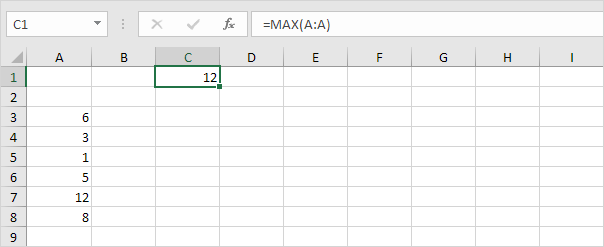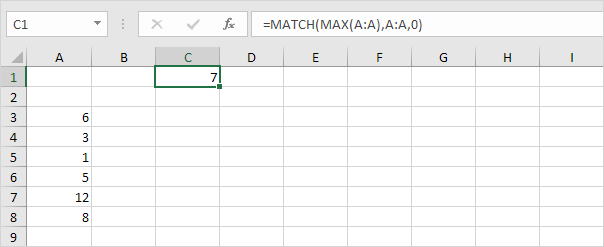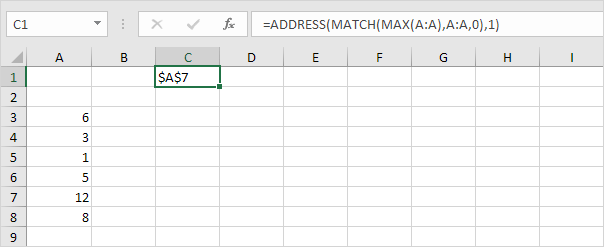# Locate Maximum Value

To find the maximum value in Excel, use the MAX function. To find the cell address of the maximum value in a column, use MAX, MATCH and ADDRESS.

1. First, we use the MAX function to find the maximum value in column A.2. Second, we use the MATCH function to find the row number of the maximum value.Explanation: the MATCH function reduces to =MATCH(12,A:A,0), 7. The MATCH function returns the position of the maximum value in column A. Set the third argument to 0 to return an exact match.

3. Finally, we use the ADDRESS function to return the cell address.Explanation: the ADDRESS function in Excel creates a cell reference as text, based on a given row and column number. The ADDRESS function reduces to =ADDRESS(7,1), \$A\$7.

Go to Next Chapter: Financial Functions